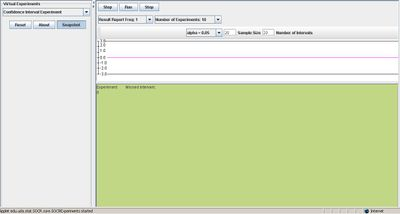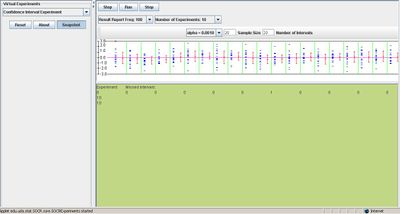# Difference between revisions of "SOCR EduMaterials Activities CoinfidenceIntervalExperiment"

## Description

This experiment randomly samples from a N(μ=0, σ2=1) distribution and constructs a confidence interval for the mean (μ). Note how confidence interval widens as the confidence level increases. This is counterbalanced by the sample-size increase. Also, see the effect of the confidence level, 100(1-α)%, on the frequency of intervals missing the estimated parameter (μ=0, in this case). A more general Confidence Intervals (CI) activity is available here.

## Goal

To provide a simple simulation demonstrating the effects of confidence level and sample-size on the size of the constructed confidence interval, and utilizing this experiment when sampling from almost all symmetric unimodal distributions.

## Experiments

Go to the SOCR Experiments and select the Confidence Interval Experiment from the drop-down list of experiments on the top left. The image below shows the initial view of this experiment:The control buttons on the top allow the user when to begin and end the experiment. “Step” runs one trial, “Run” executes a number of trials depending on the settings of the list boxes below, and “Stop” ends the trials.

The sample size and number of intervals may be modified by the user’s discretion.

Notice that when alpha has a very small value, the results are also small in value and deviation. The image below shows this information:## Applications

The Confidence Interval Experiment may be applied to any situation regarding a population parameter associated with a probability that is generated from a random sample.

For example, adjusting a machine that is to fill cans with soda so that the mean content of the cups is approximately 8 ounces. Since it is not possible for every cup to be filled at exactly 8 ounces, setting the random variable X as the weight of the filling can be applied to this applet. The distribution of X is assumed to be of a normal distribution with an expected mean value and standard deviation.

Suppose engineers want to check for the amount of pollution in the waters of Los Angeles and discover the average amount with standard deviation from the past couple of years. By using this applet, they draw generalizations of the average amount of pollution in Los Angeles with a 95% confidence level.